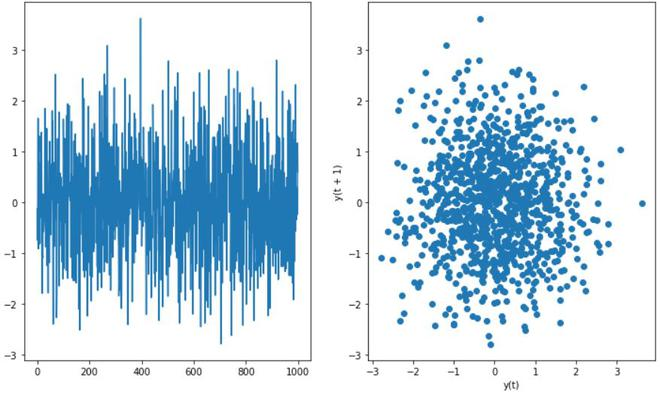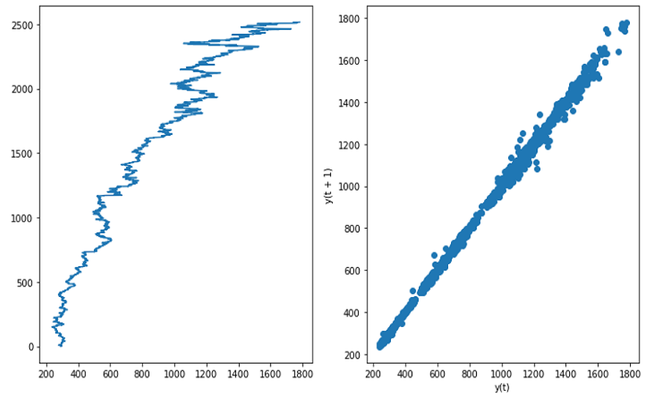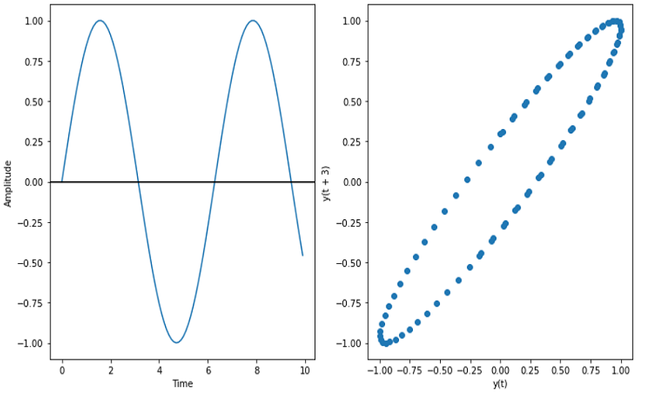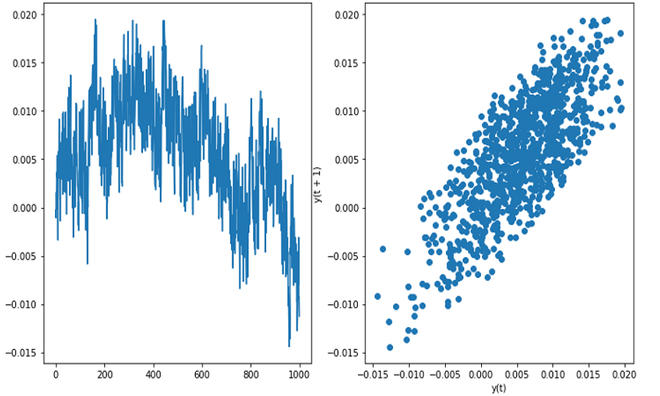Skip to content
Related Articles
Lag Plots
• Last Updated : 22 Jan, 2021

A lag plot is a special type of scatter plot in which the X-axis represents the dataset with some time units behind or ahead as compared to the Y-axis. The difference between these time units is called lag or lagged and it is represented by k.

The lag plot contains the following axes:

• Vertical axis: Yi for all i
• Horizontal axis: Yi-k for all i, where k is lag value

The lag plot is used to answer the following questions:

• Distribution of Model: Distribution of model here means deciding what is the shape of data on the basis of the lag plot. Below are some examples of lag plot and their original plot:
• If the lag plot is linear, then the underlying structure is of the autoregressive model.
• If the lag plot is of elliptical shape, then the underlying structure represents a continuous periodic function such as sine, cosine, etc.
• Outliers: Outliers are a set of data points that represent the extreme values in the distribution
• Randomness in data: The lag plot is also useful for checking whether the given dataset is random or not. If there is randomness in the data then it will be reflected in the lag plot, if there is no pattern in the lag plot.
• Seasonality: If there is seasonality in the plot then, it will give a periodic lag plot.
• Autocorrelation: If the lag plot gives a linear plot, then it means the autocorrelation is present in the data, whether there is positive autocorrelation or negative that depends upon the slope of the line of the dataset. If more data is concentrated on the diagonal in lag plot, it means there is a strong autocorrelation.

#### Implementation

• In this implementation, we will be NumPy and SciPy libraries, these are pre-installed in Colab but it can be installed in local environment using pip install. We will be using GOOGLE stock price data and Flicker data for this implementation.

## Python3

 `# Import Libraries``import` `pandas as pd``import` `numpy as np``import` `matplotlib.pyplot as plt``from` `scipy ``import` `stats as sc`` ` `# Sine graph and lag plot``time``=` `np.arange(``0``, ``10``, ``0.1``);``amplitude``=``np.sin(time)``fig, ax ``=` `plt.subplots(``1``, ``2``, figsize``=``(``12``, ``7``))``ax[``0``].plot(time, amplitude)``ax[``0``].set_xlabel(``'Time'``)``ax[``0``].set_ylabel(``'Amplitude'``)``ax[``0``].axhline(y``=``0``, color``=``'k'``)``amplitude_series ``=` `pd.Series(amplitude)``pd.plotting.lag_plot(amplitude_series, lag``=` `3``, ax ``=``ax[``1``])``plt.show()`` ` `# Random and Lag Plot``sample_size``=``1000``fig, ax ``=` `plt.subplots(``1``, ``2``, figsize``=``(``12``, ``7``))``random_series``=`  `pd.Series(np.random.normal(size``=``sample_size))``random``=``random_series.reset_index(inplace``=``True``)``ax[``0``].plot(random[``'index'``],random[``0``])``pd.plotting.lag_plot(random[``0``],lag``=``1``)``plt.show()`` ` `# Google Stock and Lag Plot (Strong Autocorrelation)``google_stock_data ``=` `pd.read_csv(``'GOOG.csv'``)``google_stock_data.reset_index(inplace``=``True``)``fig, ax ``=` `plt.subplots(``1``, ``2``, figsize``=``(``12``, ``7``))``ax[``0``].plot(google_stock_data[``'Adj Close'``], google_stock_data[``'index'``])``pd.plotting.lag_plot(google_stock_data[``'Adj Close'``], lag``=``1``,ax``=``ax[``1``])``plt.show()`` ` `# FLicker Data (Weak Autocorrelation)``df  ``=``pd.read_csv(``'Flicker.DAT'``, header``=``None``)``df.reset_index(inplace``=``True``)``fig, ax ``=` `plt.subplots(``1``, ``2``, figsize``=``(``12``, ``7``))``ax[``0``].plot(df[``'index'``],df[``0``])``pd.plotting.lag_plot(df[``0``],lag``=``1``,ax ``=``ax[``1``])``plt.show()`Random Plot (No Autocorrelation)Google Stock Data (Strong Autocorrelation)Sine Plot (elliptic curve)Flicker data (Moderate Autocorrelation)

#### References:My Personal Notes arrow_drop_up